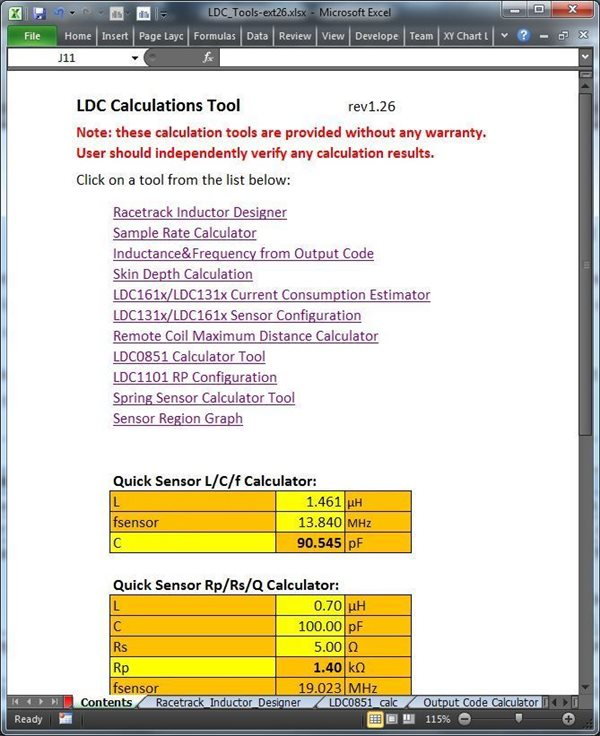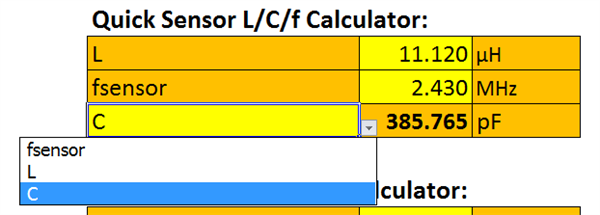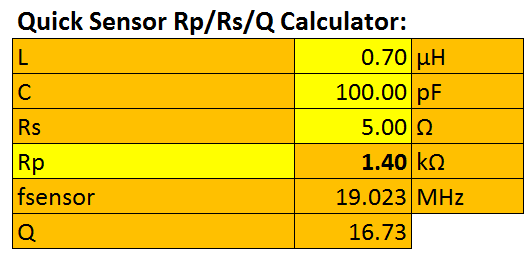# How to use the LDC calculations tool

While developing inductance-to-digital converters (LDCs) at TI, we often use a variety of spreadsheets to determine the appropriate settings or capabilities. I decided to combine all of the various tools into a single spreadsheet for convenience, and we’re releasing this spreadsheet to the web to help in your LDC system design.

The spreadsheet runs on Microsoft Excel, and you can download it here. The calculator tool doesn’t use any macros or special add-ons, so it is an easy download. While we do our best to make this tool as accurate as possible, we don’t give any warranty on the results.

The starting point for the spreadsheet tool is the Contents tab, as shown in Figure 1. This tab has a list of all the available calculation tools (see Table 1), each housed on a separate tab. Simply click the blue links to go to the appropriate calculator.Figure 1: The Contents tab of the Excel spreadsheet

To use this tool, enter your parameters in the yellow fields; the results are in the orange fields. Don’t change the formulas in the orange tabs, or else you’ll have errors or incorrect calculations.

 Calculator tab Description Racetrack Inductor Designer Designs a circular- or racetrack-shaped sensor. Sample Rate Calculator Calculates the sample rates for various LDC devices. Inductance and Frequency from Output Code Calculates sensor inductance and resonant frequency based on the device output code. Can also calculate RP from the output code for the LDC1101, LDC1000, LDC1041 and LDC1051. Skin Depth Calculation Skin depth is important for understanding performance based on target thickness and material. LDC161x/LDC131x Current Consumption Estimator Estimates the current consumption for the LDC131x and LDC161x by putting the LDC into sleep mode or shutdown mode. The application note, “Power Reduction Techniques for the Multichannel LDCs in Inductive Sensing Applications,” covers this topic in detail. LDC131x/LDC161x Sensor Configuration Calculates appropriate settings for the LDC131x and LDC161x IDRIVE. The application note, “Configuring Inductive-to-Digital Converters for Parallel Resistance (RP) Variation in L-C Tank Sensors” has more information on this topic. Remote Coil Maximum Distance Calculator LDC devices can operate when the sensor is located some distance from the LDC. This tool calculates the maximum distance for a given sensor. LDC0851 Calculator Tool Calculates the LDC0851 functionality for a given sensor, including sample rate, current consumption and estimated switching distance. LDC1101 Rp Configuration Calculates the RP_MIN, RP_MAX, T1, C1, T2 and C2 for the LDC1101. Spring Sensor Calculator Tool While mechanical engineers think that a wound-wire coil is a spring, electrical engineers know that it is really an inductor. This tool can estimate performance when using a spring as the LDC sensor.

All tabs contain a link that says Return to Main Page at the top of the tab, which will take you back to the Contents tab. See Figure 2.The Quick Sensor L/C/f Calculator is shown in Figure 3. It is a simple but very useful tool located right on the Contents tab that calculates the inductance, capacitance or oscillation frequency of an LDC sensor. (Note that this calculation doesn’t work for the LDC0851, which I’ll discuss in a future blog post.)Figure 3: Quick Sensor L/C/f Calculator on the Contents tab

First, select which parameter you wish to calculate – fsensor, L or C. Then enter the two values into the yellow fields. One nice thing about this calculator is that you can copy the 3-by-3 group of cells and paste the cells into another spreadsheet, and the copy will work independently of the original.

Right below is the Rp/Rs/Q Calculator (refer to Figure 4), which is helpful for transforming between RS and RP. It works similarly to the L/C/f calculator.Figure 4: Rp/Rs/Q Calculator

In my next post, I will cover how to use the Racetrack Calculator tool to design a sensor.